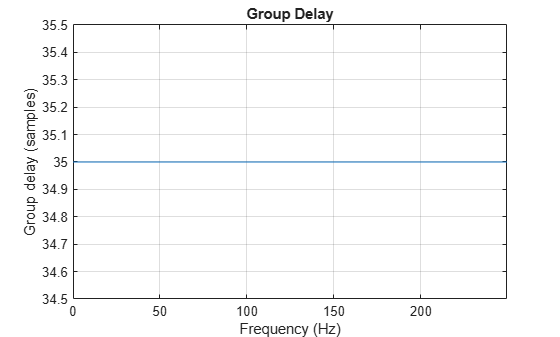# Compensate for the Delay Introduced by an FIR Filter

Filtering a signal introduces a delay. This means that the output signal is shifted in time with respect to the input. This example shows you how to counteract this effect.

Finite impulse response filters often delay all frequency components by the same amount. This makes it easy to correct for the delay by shifting the signal in time.

Take an electrocardiogram reading sampled at 500 Hz for 1 s. Add random noise. Reset the random number generator for reproducibility.

```Fs = 500; N = 500; rng default xn = ecg(N)+0.25*randn([1 N]); tn = (0:N-1)/Fs;```

Remove some of the noise with a filter that stops frequencies above 75 Hz. Use `designfilt` to design a filter of order 70.

```nfilt = 70; Fst = 75; d = designfilt('lowpassfir','FilterOrder',nfilt, ... 'CutoffFrequency',Fst,'SampleRate',Fs);```

Filter the signal and plot it. The result is smoother than the original, but lags behind it.

```xf = filter(d,xn); plot(tn,xn) hold on, plot(tn,xf,'-r','linewidth',1.5), hold off title 'Electrocardiogram' xlabel 'Time (s)', legend('Original Signal','Filtered Signal')```Use `grpdelay` to check that the delay caused by the filter equals half the filter order.

`grpdelay(d,N,Fs)``delay = mean(grpdelay(d))`
```delay = 35 ```

Shift the filtered signal to line up the data. Remove its first `delay` samples. Remove the last `delay` samples of the original and of the time vector.

```tt = tn(1:end-delay); sn = xn(1:end-delay); sf = xf; sf(1:delay) = [];```

Plot the signals and verify that they are aligned.

```plot(tt,sn) hold on, plot(tt,sf,'-r','linewidth',1.5), hold off title 'Electrocardiogram' xlabel('Time (s)'), legend('Original Signal','Filtered Shifted Signal')```